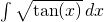## Integral of the Week #1

July 11th, 2008 | Categories: general math, Problem of the week | Tags:

I read a lot of maths related blogs, books and journals and often come across math problems that are either fun, interesting or both for one reason or another. Some I manage to solve on my own, others I solve using a CAS package like Mathematica or Matlab and then of course there are those that I solve by cheating and using google! There are also one or two that I am working on that I (or anyone else for that matter) might never solve – but I remain hopeful.

I find that solving maths problems is a lot of fun and much more satisfying than solving other types of puzzle such as crosswords or Sudoku. I am not saying there is anything wrong with these puzzles but, on the whole, I prefer to solve mathematical or computational problems.

Many other websites offer sets of problems for their readers to solve and I thought it was high time I got in on the act. Blinkdagger and Wild about Math, for example, are currently offering prizes for the solution of their problems. I will not be offering prizes for solving any of my problems but it is quite possible that you will get some warm-fuzzy feelings of pride for solving some of the tricky ones. You (and I) might even learn something too.

For the first few weeks I thought I would focus on problems from integral calculus because the solution of integrals requires techniques from many areas of mathematics such as algebra, complex analysis, numerical analysis, special functions and more. For most (but not all) of the integrals I will be featuring I know of at least one solution method already and will be posting that solution in a few weeks time. Part of the fun of integration though, is the fact that there are usually several possible solutions to any given problem and I hope to learn some new tricks and methods from you as I go along.

This week’s integral is a tricky one in my opinion (tricky if you choose not to google for it at least) but the answer can be expressed in terms of elementary functions.Solutions can be posted in the comments section or sent to me by email (obtaining my email address is another puzzle for you to solve) and will be discussed in a future post. Again, there are no prizes on offer (I am far too poor for that) but hopefully that won’t put anyone off from having a go anyway.

Update (15th July 08): If you have posted a solution in the comments and it hasn’t appeared yet it is because I am holding back for a while to allow other people to have a go. I’ll keep all comments containing solutions on hold until the 25th July.

1. Is there a u substitution that has to be done here? Trig substitution? Integration by parts?

2. I think it’s pretty straight forward. (forgive the semi-latex)
Let the integral be I=\int\sqrt{\tan x}dx

First let y=\sqrt{tan(x)}
using chain rule and the fact that \cos(\arctan a)=\frac{1}{\sqrt{1+a^2}}
we get dy=\frac{1+y^2}{2y}dx

so the integral becomes
I=2\int \frac{y^2 dy}{y^4 + 1}
=\int\left( \frac{1}{y^2+i} + \frac{1}{y^2-i} \right)dy

Then use \int\frac{dz}{z^2-b^2}=-\frac{1}{b}\arctanh\frac{z}{b}
to get the solution (remembering y=\sqrt{tan(x)} )
I = – (-1)^{1/4} \arctanh((-1)^{1/4} y) – (-1)^{-1/4} \arctanh((-1)^{-1/4} y)

it may be “simplified” by splitting the arctanh’s as
\arctanh(z)=\frac{1}{2}( \log(1+x)-\log(1-x) )
and the \sqrt{i}’s as
(-1)^{\pm 1/4}=\frac{1}{\sqrt{2}}(1 \pm i)
then recombining. Being lax with the log’s branch cuts we can write
I=\frac{1}{2\sqrt2}\left(
\log\frac{1-\sqrt{2}y+y^2}{1+\sqrt{2}y+y^2} + i (y\to\i y) \right)

Taking the derivative of this expression wrt x in mathematica yields the original integrand. :)

3. Nice one simon :)